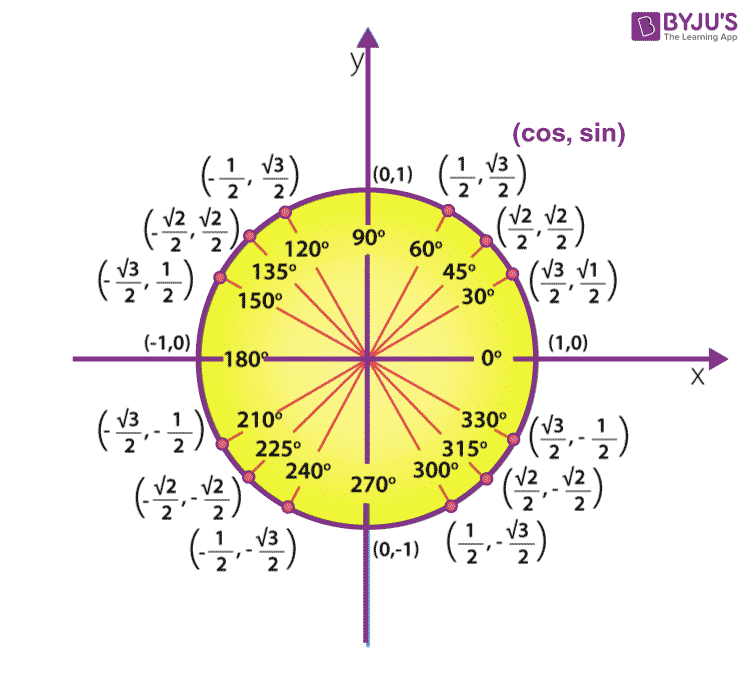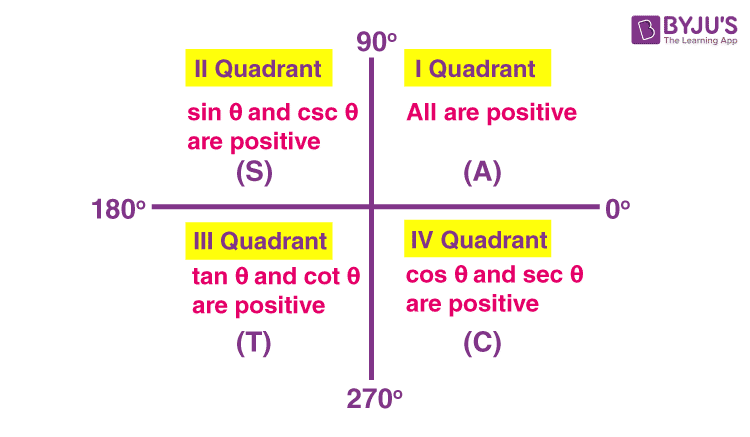# Tan 180

In trigonometry, the value of tan 180 (in degrees) is equal to 0. This value can be obtained using trigonometry ratios of complementary angles. The trigonometric Table of sin, cos, tan, cosec, sec, and cot is helpful to determine the standard angles of trigonometric ratios from 0° to 360°. We can apply different formulas in trigonometry to get the required values for trigonometric functions. In this article, you will learn what the tan 180 value is, how to find the value of tan 180° with solved examples.

## Tan 180 Value

Tan 180 value (in degrees) is given as:In a circular system, tan 180° can be written as tan π.

As we know the tan can be expressed as sin over cos. This relation helps in deriving the tan 180 value from the unit circle.

 Read more:

For a unit circle, which has a radius equal to 1, we can derive the tangent values of all the degrees. With the help of a unit circle drawn on the XY plane, we can find out all the trigonometric ratios and values.In the above figure,

sin 180° = 0 and cos 180° = -1

Now,

tan 180° = sin 180°/cos 180° = 0/(-1) = 0

Also, get the trigonometric functions calculator here to find the values for all the angles.

## Tan 180 Degrees

In this section, you will learn how to derive the value of tan 180 degrees in different methods.

Method 1:

Before deriving the tan 180 value, let us recall the complementary angles formulas in trigonometry so that we can easily understand this method of derivation.

• sin (90° – A) = cos A
• cos (90° – A) = sin A
• tan (90° – A) = cot A
• cot (90° – A) = tan A
• sec (90° – A) = cosec A
• cosec (90° – A) = sec A
• sin(90° + A) = cos A
• cos(90° + A) = -sin A
• tan(90° + A) = -cot A

The below figure shows the sign of trigonometric functions in different quadrants.Now, tan 180° can be written as:

tan 180° = tan(90° + 90°)

From the above figure, we can say that tan(90° + A) lies in the second quadrant, where tan is not positive.

= -cot 90°

= -(cos 90°/sin 90°)

= -(0/1)

= 0

Therefore, tan 180° = 0

Or

tan 180° = tan(180° – 0) = -tan 0° = 0 {since tan 0° = 0}

This value is taken from the trigonometry table.

Method 2:

In this method, we directly substitute the values of sin 180° and cos 180° from the table values of trigonometry ratios in the tan formula.

tan 180° = sin 180°/cos 180° = 0/(-1) = 0

## Tan 180 – Theta

Let us derive the formula for tan 180 – theta, i.e. tan(180° – θ).

tan(180° – θ) = tan[2 × 90° – θ]

Here, 90° is multiplied by an even number 2. So the trigonometric function will not change. That means, in the next step we should write “tan” only.

Also, 180° – θ lies in the second quadrant, where tan is not positive (only sine and cosecant are positive).

Thus, tan(180° – θ) = -tan θ

Similarly, tan(180° – x) = -tan x

And

tan (180° – a) = -tan a

All these formulas can also be expressed as:

tan(π – θ) = -tan θ

tan(π – x) = – tan x

tan(π – a) = -tan a

### Solved Examples

Example 1: Find the value of tan 120°.

Solution:

tan 120° = tan(180° – 60°)

= -tan 60°

= -√3

Example 2: Evaluate: 2 tan 180° – tan 150°

Solution:

2 tan 180° – tan 150°

= 2 tan 180° – tan(180° – 30°)

= 2(0) – (-tan 30°)

= 0 + tan 30°

= 1/√

## Frequently Asked Questions on Tan 180

### What is the value of tan 180 degrees?

The value of tan 180 degrees is 0, i.e. tan 180° = 0.

### What is sin 90 value?

The value of sin 90 degrees is equal to 1.

### What is the formula of tan 180 – Theta?

The formula for tan 180 – theta is given as:
tan(180° – θ) = -tan θ
Or
tan(π – θ) = -tan θ

### What is tan 45 value?

The value of tan 45 degrees is equal to 1. This value can be calculated as:
tan 45° = sin 45°/cos 45°
= (1/√2)/ (1/√2)
= 1

### How do you find COT 30 degrees?

The value of cot 30 degrees can be calculated as:
cot 30° = 1/tan 30° = 1/(1/√3) = √3